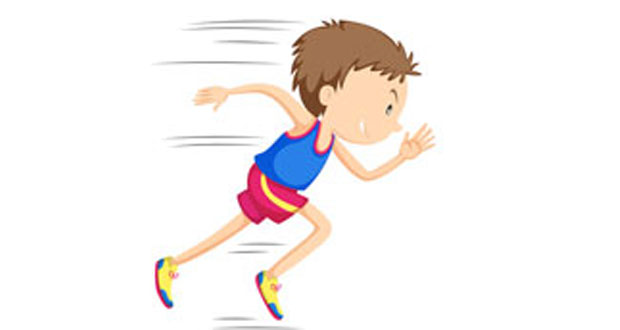# Linear Motion & BiomechanicsLinear motion simply means motion in a straight line (as opposed to circular motion or rotation). In order to talk about linear motion scientifically, we need to be familiar with mass, distance, displacement, speed, velocity, and acceleration. Here we explain Newton’s laws of motion, mass, inertia, momentum, speed, velocity, distance, displacement, and graphs of motion.

## Speed and Velocity

Here we explain the difference between distance and displacement, vector and scalar quantities as well as speed and velocity in sport and how to do calculations. What is the difference between distance and displacement? Displacement is how far away a body has moved from its starting point.

## Acceleration

Acceleration is the rate at which a body changes its velocity and, similarly to velocity, it is a vector quantity which means it has a direction as well as a magnitude. It is measured in m/s/s or metres per second per second (metres per second squared – ms-2).

## Newton’s Laws of Motion

Sir Isaac Newton stated three laws that explain the relationship between force and movement. Having a good understanding of Newton’s laws, which can be applied to sporting situations, will allow you to gain a deeper understanding of sports techniques.

## Graphs of motion

Graphs are a good way of showing motion visually. The two types of graphs that are of most interest are distance/time graphs and speed/time (or velocity/time) graphs. Graphs are particularly useful when things are moving too fast for the naked eye and brain to understand what is happening, which is often the case in sports.

## Mass, Inertia, and Momentum

Inertia is the reluctance of a body to change whether it is moving or not and is related to its mass. Once a body is moving the momentum of the body is a product of its mass multiplied by its velocity.